# Dessins d’Enfants

## Mathematician Gareth Jones on compact Riemann surfaces, algebraic numbers and what is the absolute Galois group

### videos | September 10, 2020

Dessins d’enfants is a French phrase, it means children’s drawings. It’s a theory that was developed by Grothendieck in the 1980s, and he was fascinated by the fact that very simple drawings such as one I will show you now can encode very sophisticated mathematical information. Here is an example: this is the sort of drawing which a child might make to represent a human being. It’s sometimes called the one-armed bandit or the matchstick man.

What it represents is a Riemann surface of genus 3601, an algebraic curve defined by a polynomial of degree 12 with coefficients in a certain algebraic number field. The automorphism group of this Riemann surface is the simple group M12, the Mathieu group of order 95040.

So this very simple drawing, just a few points on lines on a sheet of paper, represents some very complicated mathematics. That was why Grothendieck gave this rather strange name, dessins d’enfants, ‘children’s drawings’ to this theory.

To explain this theory we have to go back 160 years to Riemann. In the middle of the 19th century Riemann introduced what we now call Riemann surfaces. These are surfaces like the sphere or the torus or surfaces of higher genus in which locally you have complex coordinates. This allows you to imagine, locally at least, that you are in the complex plane so you can do calculus, you can differentiate, you can integrate and so on. So you can do calculus, you can do analysis, and locally it works just as in the complex plane but globally, depending on the shape of the surface, the answers may be rather different.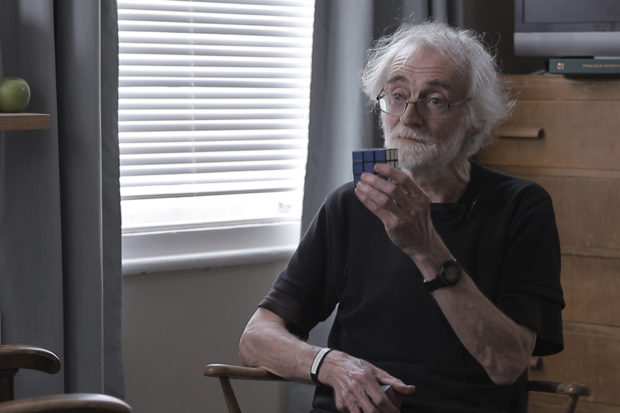Mathematician Gareth Jones on abelian and non-abelian groups, the symmetry of geometric objects and what are the principles a group must satisfy
What Riemann proved was that compact Riemann surfaces (these are essentially those with finite area) are equivalent to complex algebraic curves. These are varieties defined by polynomial equations with complex coefficients which are one-dimensional over the complex numbers. There’s a possible confusion about names here: I’ve called them Riemann surfaces and I’ve called them algebraic curves. Surfaces are two-dimensional; curves are one-dimensional. Why this confusion? Well, these surfaces are one-dimensional over the complex numbers but the complex numbers form a plane, and that is two-dimensional. So it is simply a change of name: one lot of mathematicians call them surfaces, the other lot call them curves. They are essentially the same object.

Now, because these compact Riemann surfaces can be defined by polynomial equations, it’s of interest to mathematicians to know for particular subfields of the complex numbers which Riemann surfaces can be defined over those subfields, that is by polynomials with coefficients in those fields. For some subfields, such as the real numbers, the answer is easy; for others, such as the field of rational numbers, it appears to be extremely difficult. There’s an intermediate case which concerns the field of algebraic numbers.

The algebraic numbers are those complex numbers which can be found as roots and polynomials with integer coefficients. They include all the rational numbers, some irrational numbers such as the cube root of 2, some imaginary numbers such as the square root of -1, but they do not include transcendental numbers like e or π which have to be defined using geometry or analysis. These algebraic numbers form a field, they’re closed under addition, subtraction, multiplication and division.

The question then is which Riemann surfaces are defined using algebraic numbers? This is the question which Grothendieck was wrestling with in the early 1980s. And then he discovered that a Russian mathematician called Belyi had already answered the question in a lemma which was tucked away in a paper on a different subject, namely Galois theory. What Belyi did was he showed that the Riemann surfaces defined over algebraic numbers can be characterised as having a meromorphic function on them which is branched over at most three points. That’s a very technical condition but Grothendieck was able to reinterpret this condition to show that the Riemann surfaces defined over algebraic numbers are precisely those which can be obtained from maps on surfaces, that is graphs drawn on surfaces like the little example I showed you.

Now, how do you obtain a Riemann surface from such a graph drawn on the surface? Every such map is a quotient of what is called the universal map: this is an infinite map drawn on the hyperbolic plane. The hyperbolic plane is a Riemann surface, it’s a subset of the complex numbers, so it has the structure of a Riemann surface, and every quotient of it therefore inherits the structure of a Riemann surface. So these quotients are themselves Riemann surfaces. In fact, they are compact Riemann surfaces and the ones that you obtain in this way are precisely those which are defined over the field of algebraic numbers.

These little drawings give you an exact characterisation of the Riemann surfaces and the algebraic curves which Grothendieck was investigating at that time.

Already that was a remarkable result that you can describe these very complicated structures by simple drawings like that.

But he did rather more than that because Grothendieck was interested in studying what is called the absolute Galois group. This is the automorphism group of the field of algebraic numbers. It’s a very big group; in fact, it’s uncountable, so it’s certainly not finitely generated. It’s complicated, it has a topology, it’s a topological group, but it has the topology of a Cantor set which is very pathological: it’s not smooth in any reasonable sense, it’s a very fractured group. It’s an important group because in a certain sense it encodes the whole theory of algebraic number fields.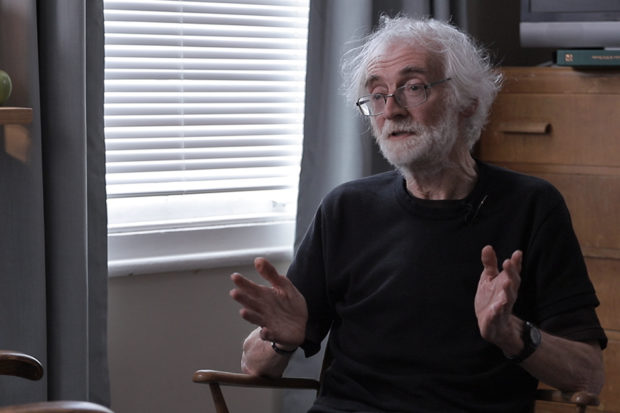Mathematician Gareth Jones on Gödel's incompleteness theorem, the halting problem and why the subsets of the natural numbers are uncountably infinite
So if we understand this group, we essentially understand the whole of algebraic number theory and we would be able to solve many problems in that area. For example, Hilbert conjectured that every finite group can be realised as a Galois group of an algebraic number field. This problem is still open. It was proved for some groups, for example, solvable groups by Shafarevich a few decades ago but there are some other groups for which the question is still open. It’s equivalent to showing that this absolute Galois group has among its quotients biclosed normal subgroups or finite groups, so if we understood this absolute Galois group and its quotients, we could answer questions like this question of Hilbert’s.

So it’s very important to understand this group but it’s a very difficult group to work with. If we can find something for this group to act on, then it will give us some insight into what this group looks like and its internal structure. These maps on surfaces give us a geometric way of seeing the internal structure of this absolute Galois group. How does that work? The absolute Galois group consists of the automorphisms of the field of algebraic numbers, so the elements of this group permute the algebraic numbers, they change them around. They therefore can be applied to the coefficients of the polynomials defining these compact Riemann surfaces, these complex algebraic curves.

So an element of the absolute Galois group can be applied to these coefficients and it can convert one polynomial into a different polynomial. It can therefore change the algebraic curves, and therefore it can change the dessins d’enfants, the maps associated with them.

So you can see the elements of the absolute group in action by the way they transform these dessins into each other. As a very simple example, let’s go back to the example I showed you at the beginning. Complex conjugation is an element of the absolute Galois group. It’s the easiest non-identity element to see. What does it do to any dessin d’enfants? Well it’s not difficult to guess: it acts by transforming it to its mirror image. That’s a rather trivial example, there are much more complicated examples where elements of the absolute Galois group act in a much more dramatic way transforming one dessin into another one.

This has led to a great theory in which mathematicians have tried to elaborate the ideas put forward by Grothendieck. Grothendieck wrote a great set of notes ‘Esquisse d’un Programme’ (Sketch of a program) in 1984 and then more or less gave up mathematics. He lived the last few decades of his life as a hermit in the Pyrenees with almost minimal contact with the rest of the world. He died a few years ago but he left us this great body of work full of conjectures, full of ideas, and in the decades since then mathematicians have been filling in the details of Grothendieck’s theory. There’s still a long way to go; we don’t understand the orbits of the absolute Galois group, we can’t determine whether two dessins are equivalent under the absolute Galois group but we’re working on it, so there’s plenty more work to do.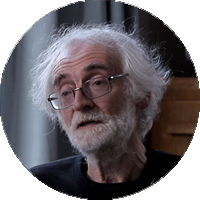Emeritus Professor of Mathematics, University of Southampton
Did you like it? Share it with your friends!
Published items
0779
To be published soon
+92

#### Most viewed

• 1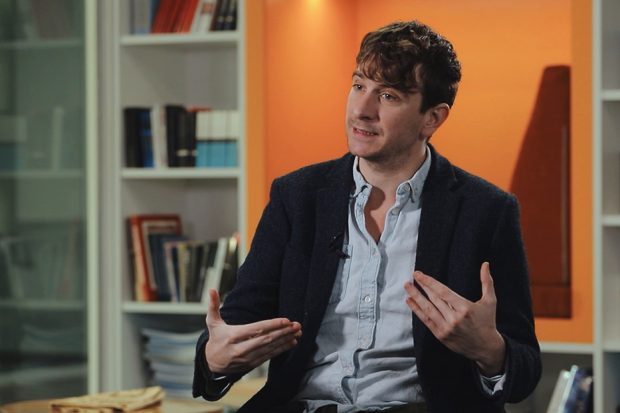Peter Jones
• 2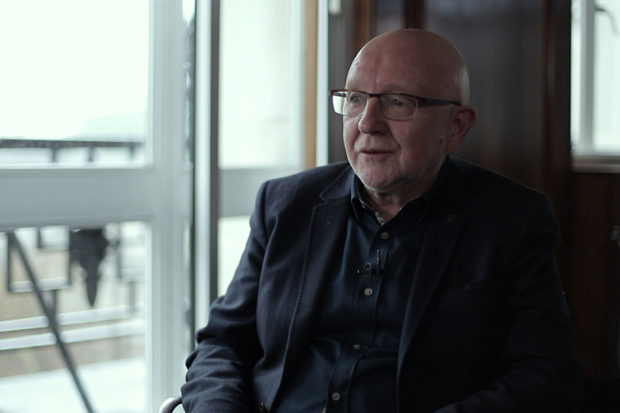John Worrall
• 3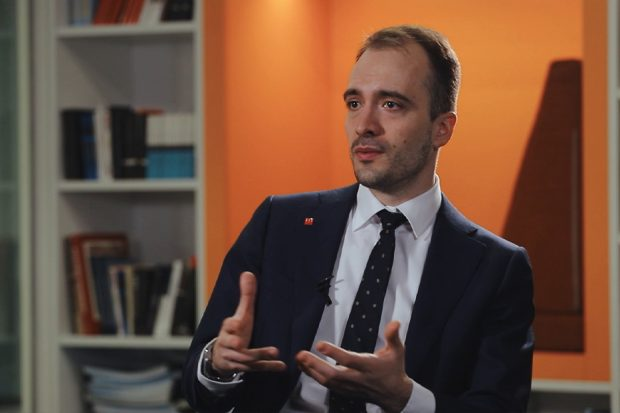Daniel Kontowski
• 4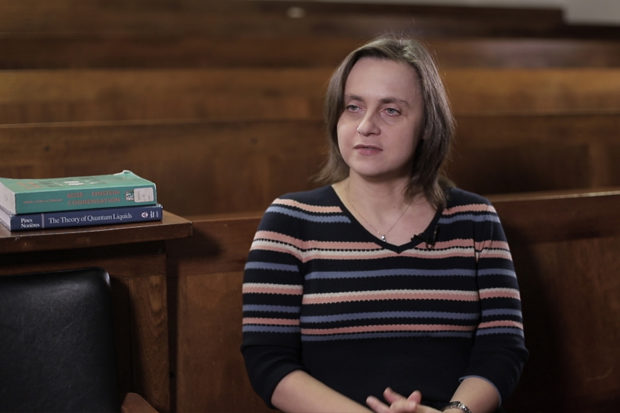Marzena Szymanska
• 5Jonathan Birch
• 6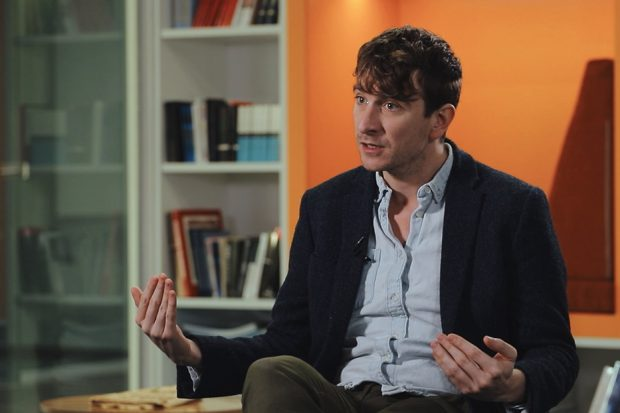Peter Jones
• 7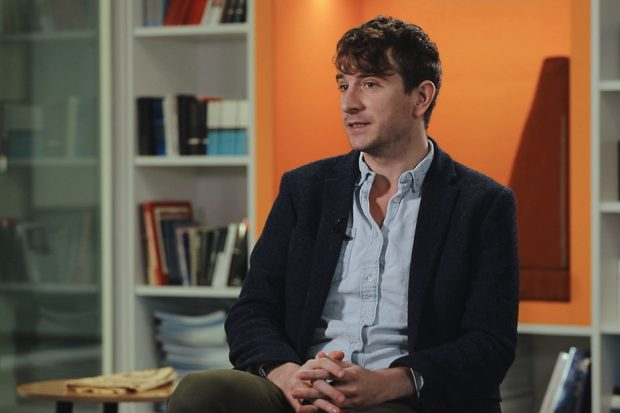Peter Jones
• 8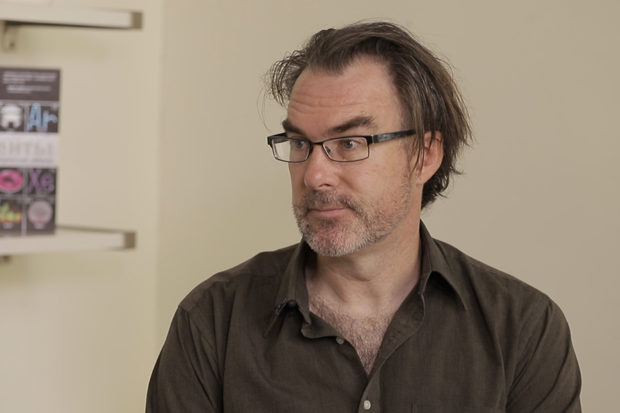Jeffrey Taylor
• 9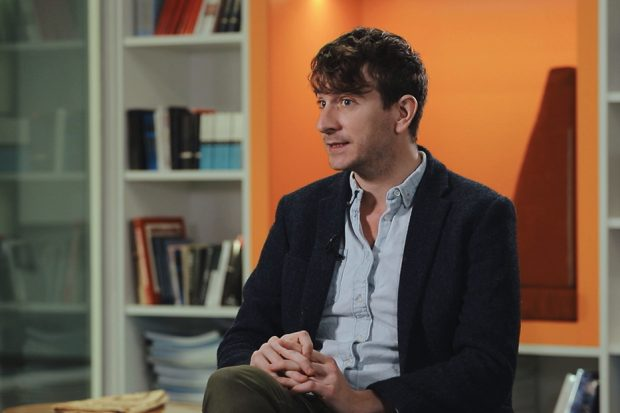Peter Jones
•